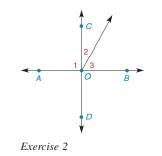Chapter 1.6, Problem 2E### Elementary Geometry for College St...

6th Edition
Daniel C. Alexander + 1 other
ISBN: 9781285195698

#### Solutions

Chapter
Section### Elementary Geometry for College St...

6th Edition
Daniel C. Alexander + 1 other
ISBN: 9781285195698
Textbook Problem
1 views

# In Exercise 1 and 2, supply reasons. Given: A B ↔ intersects C D ↔ at O so that ∠ 1 is a right ∠ (Use the figure following Exercise 1.) Prove: ∠ 2 and ∠ 3 are complementary. PROOF Statements Reasons 1. A B ↔ intersects C D ↔ at O . 1. ? 2. ∠ A O B is a straight ∠ , so m ∠ A O B = 180 2. ? 3. m ∠ 1 + m ∠ C O B = m ∠ A O B 3. ? 4. m ∠ 1 + m ∠ C O B = 180 4. ? 5. ∠ 1 is a right angle 5. ? 6. m ∠ 1 = 90 6. ? 7. 90 + m ∠ C O B = 180 7. ? 8. m ∠ C O B = 90 8. ? 9. m ∠ 2 + m ∠ 3 = m ∠ C O B 9. ? 10. m ∠ 2 + m ∠ 3 = 90 10. ? 11. ∠ 2 and ∠ 3 are complementary 11. ?To determine

To prove:

That 2 and 3 are complementary and give the reasons in the table form.

Explanation

Given:

The line AB intersects CD at O so that 1 is a right angle.

And,

The given figures are,

Figure (1)

Figure (2)

The given table is shown below.

 PROOF Statements Reasons 1. AB↔ intersects CD↔ at O. 1. ? 2. ∠AOB is a straight ∠, so m∠AOB=180° 2. ? 3. m∠1+m∠COB=m∠AOB 3. ? 4. m∠1+m∠COB=180° 4. ? 5. ∠1 is a right angle 5. ? 6. m∠1=90° 6. ? 7. m∠1+m∠COB=180° 7. ? 8. m∠COB=90° 8. ? 9. m∠2+m∠3=m∠COB 9. ? 10. m∠2+m∠3=90° 10. ? 11. ∠2 and ∠3 are complementary 11. ?

Property used:

a. The Transitive Property of Congruence states that if two angles are each congruent to a third angle, then they are congruent to each other.

b. The Additive Property of Equality states that if same number is added to both sides of the equation then the sides of the equation remains equal.

c. If the measure of two angles is equal then the angles are known as congruent angles.

d. According to Substitution Property, if x=y, then x can be substituted for y and y can be substituted for x in any equation.

e. A straight angle has measure 180°.

Approach:

The first statement of the proof is given. That is.

The line AB intersects CD at O.

The proof should always be given, so the reason for the first statement is “Given”.

The second statement of the proof is given. That is.

mAOB=180°

From the given figure it can be observed that the angle AOB is a straight angle so, the measure of AOB is 180° so, the reason for the second statement is “The measure of a straight angle is 180°”.

The third statement of the proof is given. That is.

m1+mCOB=mAOB

In the third statement C is the interior of AOB so, according to Angle-Addition Postulate the sum of 1 and COB is equal to AOB.

So, the reason for the third statement is “Angle-Addition Postulate”.

The fourth statement of the proof is given. That is.

m1+mCOB=180°

Substitute the value from the second statement to third statement.

So, the reason for the fourth statement is Substitution Property.

The fifth statement of the proof is given. That is “1 is a right angle”

### Still sussing out bartleby?

Check out a sample textbook solution.

See a sample solution

#### The Solution to Your Study Problems

Bartleby provides explanations to thousands of textbook problems written by our experts, many with advanced degrees!

Get Started

#### Place the following set of n = 20 scores in a frequency distribution table.

Essentials of Statistics for The Behavioral Sciences (MindTap Course List)